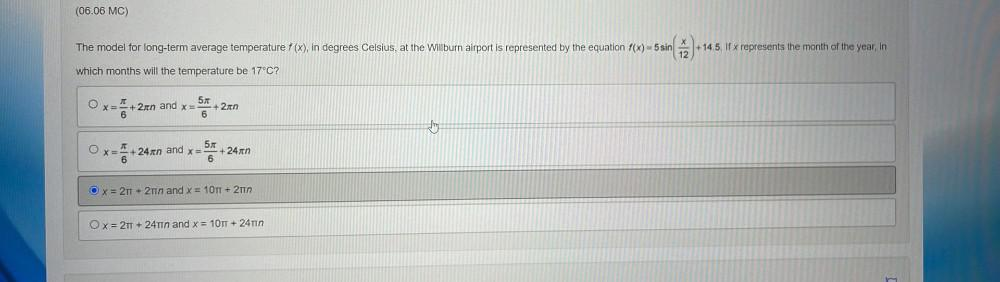Question:

# (06.06 MC) X The model for long-term average temperature f (x), in degrees Celsius, at the Willburn airport is represented by th(06.06 MC) X The model for long-term average temperature f (x), in degrees Celsius, at the Willburn airport is represented by the equation f(x) = 5 sin +14.5. If x represents the month of the year, in 12 which months will the temperature be 17°C? JT 57 x= + 2n and x= + 2n 6 6 Thing O 57 x= +24gen and x= +24xn 6 6. = Ox=2 TT + 2 and x = 10TT + 2T Ox= 2T + 24T1n and x = 10TT + 24TIN 3